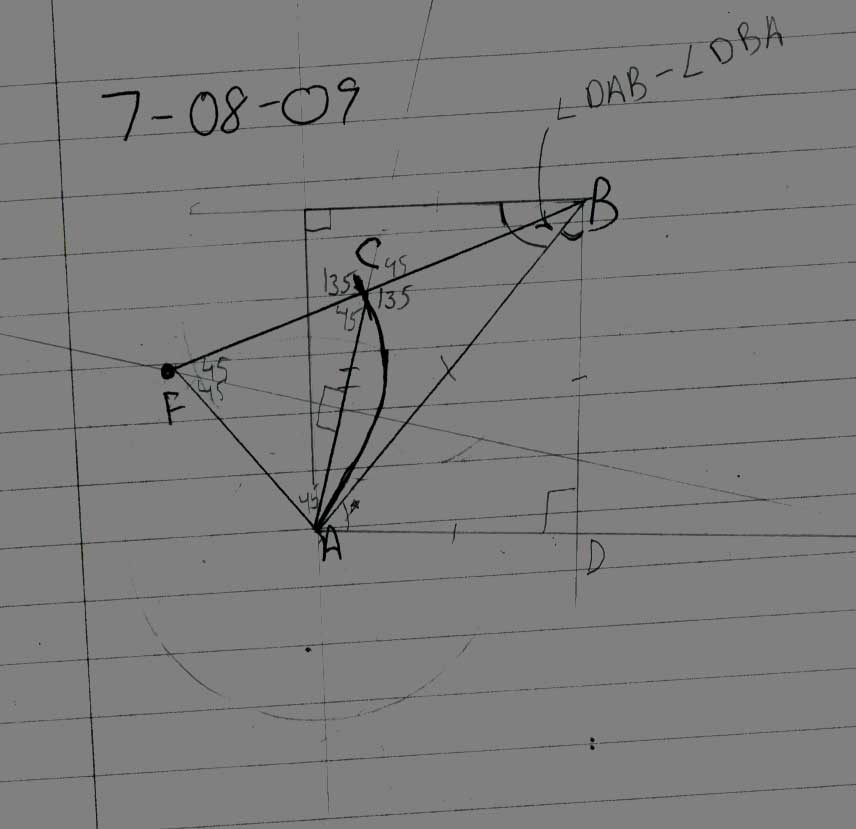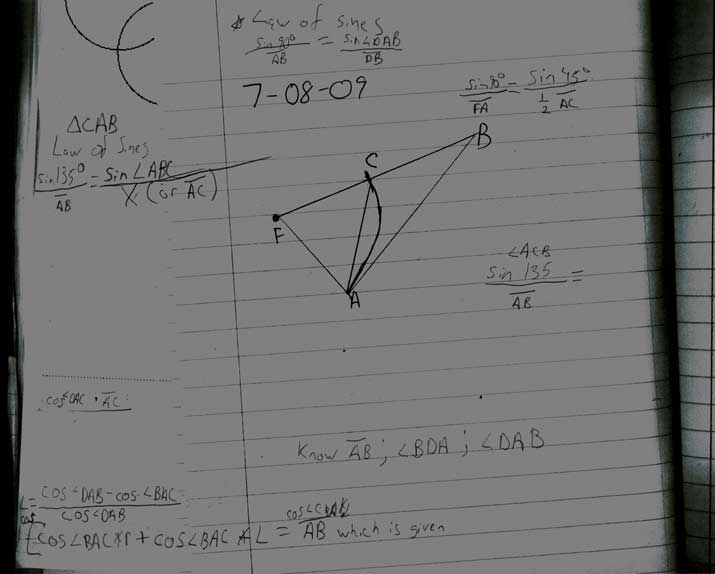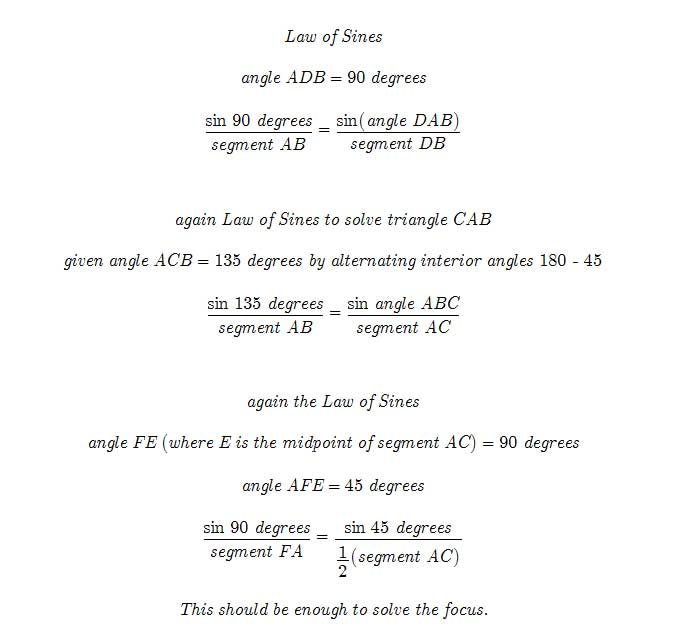_______
20090708-0709

So we continue our mathematical journey to solve a parabola only knowing 2 points. This may however not solve for just one unique parabola. It must be tested. But as the chosen points distance increases towards infinity the probability of finding a unique parabola increases.

We are given 2 points and connect them with a line. We’ll call it line AB. Then we try to determine the focus of the parabola. To help us we construct triangles as seen in the diagrams. We draw segment FA. If we visualize this to be a radius that draws out a circle with point C. This gives us a 90-45 degree triangle.

Follow the following equations to solve for the focus. It is very basic. It is based on the Law of Sines.

I just hope this work!

May the Creative Force be with You